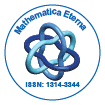# Mathematica EternaOpen Access

ISSN: 1314-3344

## Abstract

### 3-1 Piecewise NCP Function for Nonlinear Complementary Problem

Ke Su and Dan Yang

In this paper, we define a 3-1 piecewise linear NCP function and proposed a modified nonmonotone method for nonlinear complementarity problem. Then, we use a piecewise NCP function to transform the original problem into a semi-smooth equation. This algorithm solves a system of linear equations with the nonsingular coefficient matrix and introduces a nonmonotone linear search. Under some suitable assumptions,we prove the globally convergence of the algorithm.

Top